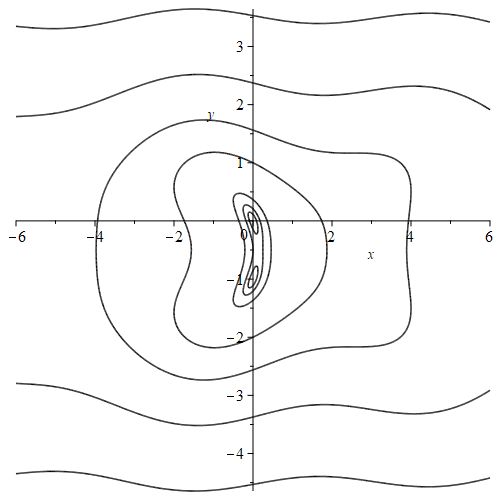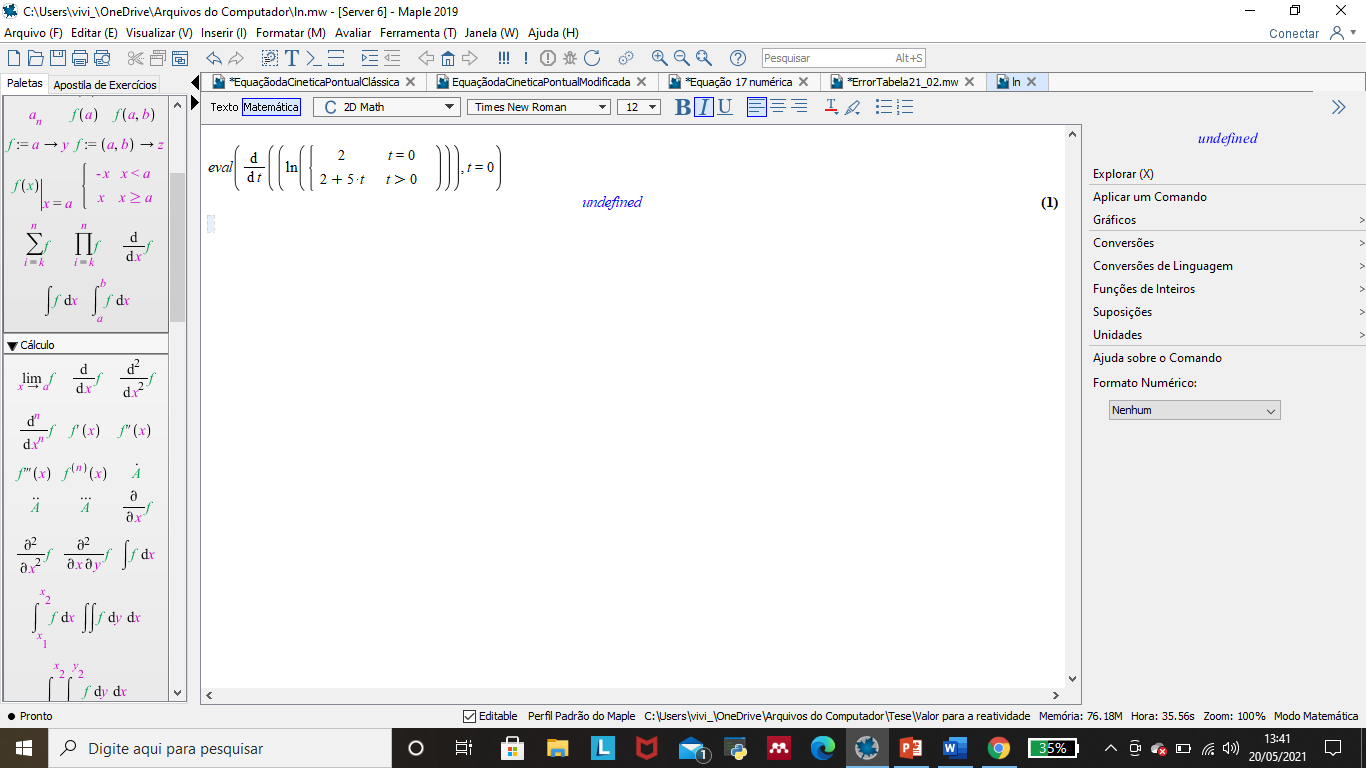## what solver PDEtools:-Solve uses by default...

I noticed PDEtools:-Solve hangs sometimes on some input where solve does not. When I change the solver for PDEtools:-Solve to use solve, it did not hang.

This means PDEtools:-Solve default solver is something else. I did not see what it is by looking at help.

Here is an example

```restart;
eq:=[1/3*(-334*I*(-4807763*I+27000*I*2^(1/2)+60*(1746675+72116445*2^(1/2))^(1/2))^(1/3)-2*(-4807763*I+27000*I*2^(1/2)+60*(1746675+72116445*2^(1/2))^(1/2))^(2/3)+56978)/(-4807763*I+27000*I*2^(1/2)+60*(1746675+72116445*2^(1/2))^(1/2))^(1/3)*v-20*I*2^(1/2)*v = 0, (-18000*I*2^(1/2)+33400*I-40*(1746675+72116445*2^(1/2))^(1/2)-40*(-167*I*(1746675+72116445*2^(1/2))^(1/2)+75150*2^(1/2)+10*(-4807763*I+27000*I*2^(1/2)+60*(1746675+72116445*2^(1/2))^(1/2))^(2/3)+145445)/(-4807763*I+27000*I*2^(1/2)+60*(1746675+72116445*2^(1/2))^(1/2))^(1/3))/(167*I*(-4807763*I+27000*I*2^(1/2)+60*(1746675+72116445*2^(1/2))^(1/2))^(1/3)+(-4807763*I+27000*I*2^(1/2)+60*(1746675+72116445*2^(1/2))^(1/2))^(2/3)-28489)*v-20*I*2^(1/2)*t = 0];

solve(eq,[v,v]); #OK
PDEtools:-Solve(eq,[v,v],solver=solve); #OK
PDEtools:-Solve(eq,[v,v]); #hangs
```

Maple 2021

## solving a system of trigonometric equations...

Hey guys I need help solving the following system of trigonometric equations

`invEqs := [a*cos(theta + theta + theta) + a*cos(theta + theta) + a*cos(theta) = px, a*sin(theta + theta + theta) + a*sin(theta + theta) + a*sin(theta) = py, theta + theta + theta = phi]`

which everything except theta, theta, theta are known.

here what I came up with but no success

`res := solve(invEqs, {theta, theta, sin(theta)})`

or better be like this but no output printed

`res := solve(invEqs, {theta, theta, cos(theta)})`

## Power series solution of nonlinear ODE...

How to find the power series solution of a nonlinear 4th order ordinary differential equation.

>ode:=U""+c^2*U"+k*c*U"-(3U^2+a)*U''=0

## HUE shading for implicit function...

hi! is there any kind of formula for coloring functions by HUE like this

contourplot(abs(sin(x) + y^2 + y + x*I), x = -6 .. 6, y = -5 .. 5, contours = [seq(1/2^n, n = 1 .. 3), seq(2^n, n = 1 .. 5)], numpoints = 200000, thickness = 1.5, color = black);I wonder about situation when we don't have function for example f(z)=z but f(x,y)=x+I*y and we want to use domain coloring for that. Thanks in advance.

## How do I solve a equation ln in Maple?...

Hi!

How do I solve a equation ln in Maple:ln.mw## How to compute te expectation of an abstract rando...

Let E be a random variable of expectation mu and A an algebraic expression containing no random variable.
If E has any known Maple distribution, then  Mean(A+E) = A+mu.

But if E is an "abstract" random variable, Mean doesn't seem capable to compute the expectation of A+E.
Notional example:

```restart:
with(Statistics):
E := RandomVariable(Normal(mu, sigma)):
Mean(f(x)+E)
f(x) + mu
E := RandomVariable(Distribution(PDF = (z -> f(z)), Mean=mu)):
Mean(f(x)+E);
int((f(x) + _t) f(_t), _t = -infinity .. infinity)

IntegrationTools:-Expand(%);
f(x) (int(f(_t), _t = -infinity .. infinity)) + (int(f(_t) _t, _t = -infinity .. infinity))
```

Questions:

• Why does Mean not behave as expected for an abstract random variable?
• Is there a simple way to obtain the expected result (Mean (A+E) = A+mu) (maybe by completing the definition of the distribution of E, or by any other means)?

TIA

PS: I know that I can replace Mean(A+E)  by A+Mean(E)  to obtain the desired result: this is not the type of answer I look for.

PS: I know (since Carl Love showed me how long ago) that I can define a "random variable" plus an operator Expectation such that Expectation(A+E)  by A+Expectation(E) ... but it's not a way I would call simple

```Expectation := proc(e::algebraic)
local a,b;
if not hastype(e, RV) then e
elif e::RV then 'procname'(e)
elif e::`+` then map(thisproc, e)
elif e::`*` then
(a,b):= selectremove(hastype, e, RV);
b*thisproc(a)
else 'procname'(e)
end if
end proc:

#------------------------------------------------------------------------

RV,
{RandomVariable,
'RandomVariable^posint',
'`*`'({RandomVariable, 'RandomVariable^posint'})
}
):
eval(Expectation(f(x)+E), Expectation=Mean)
f(x) + mu
```

## How to run this: ` Computes A*B mod b^d by convolu...

13.5.2021

 >>
 >
 >>>>>>>>(1)
 >>Hi 😊

I have found this Code here somewhere in MaplePrimes.

I am very interested in that Procedure, but it doesn't run.

I will be very happy, to get help with that.

Thanks a lot,

Arno

## How to output "(mol/L)^2" instead of "L^2/mol^2"?...

Hello

When doing chemistry in Maple, I want to be able to do my calculations with units.

Maple does not support the unit [M] (molar concentration), so I have to manually type the unit as [mol/L].

That is not a problem in itself, but I have encountered som issues with Maple's own formatting of the units.

For example, in the attached document I want the output unit to be (mol/L)^(-2). However, Maple automatically formats it asIt even does the same thing in the "Maple Math" here on MaplePrimes - Here I try to type (mol/L)^(-2):So does anyone know a way to avoid Maple's auto-formatting of the output, or is there a way to customize it so I get my desired output?

I have attached the document with some screenshots in it: chemistry_units.mw

// Frederik

## typematch behaving differently after/before copy a...

primes.mw

As a result of a calculation I have a long expression. I give it a name, say, X,

X:= long expression.

I try to use typematch to extract different parts of X and its does not work (it returns false).

If, however, I copy and paste the long expression from the output and plug into my typematch query it works!

It seems that copy and paste change the type of the expression. I am very confused with this.

I have uploaded a file with the problem at the beggining.  Thank you!
Max

## Multi Matrix multiplication...

Hey guys, how can I apply multiplication to a sequence of matrices like A, A...A[n] so it becomes A*A...*A[n] ? thanks in advance

## How can I play a tone in Maple?...

I am investigating the history of scales and temperament in western music. I would like to be able to play a tone of a given frequency in Maple, and then a sequence of tones to make a scale, and then a collection of tones to make a chord. I found the Play command  in the online help screen for Audio Tools, but can't make it work. I don't get an error message, but neither do I get a sound.

## how to use uses between child modules? ...

I am merging two separate modules  B,C to be child modules inside one main A module.

Now, I found the code breaks, because uses does not work any more.

Before merging, C module did uses B. But now this gives an error when both B,C sit inside one parent module.  An example make this easier to explain

```restart;
interface(warnlevel=4);
kernelopts('assertlevel'=2):

A:=module()

export B:=module()
export foo:=proc()
print("In B:-foo()");
end proc;
end module;

export C:=module()
uses B; #this cases problem
export boo:=proc()
foo();
end proc;
end module;
end module;

Error, (in A:-C) no bindings were specified or implied
```

Changing uses B; to uses A:-B; does not help. I get error Error, (in A:-C) `A` does not evaluate to a module

I also tried uses :-A:-B; , now this does not give error, but it does not work. i.e. when doing  A:-C:-boo() Maple does not end up calling foo() inside module B as expected.

One way to avoid all this, is not to use uses B inside the module and do this instead

```A:=module()

export B:=module()
export foo:=proc()
print("In B:-foo()");
end proc;
end module;

export C:=module()
export boo:=proc()
B:-foo();
end proc;
end module;
end module;```

But it means I have to now change lots of code inside the C module, and add an explicit B:- everywhere

This is how it was before the merging

```B:=module()
export foo:=proc()
print("In B:-foo()");
end proc;
end module;

C:=module()
uses B;    #no problem now
export boo:=proc()
foo();  #this now uses B:-foo() automatically due to uses.
end proc;
end module;
```

The above works. Now I can do C:-boo() and it works as expected.

The question is: How to make it work after moving both C and B inside one parent module so I do not have to change lots of code? I tried many things, but can't get it to work.

Maple 2021 on windows 10

Edit

Thanks for the answers below. I think I have to change my code then either way to add a prefix to the call. I actually always use the long form of the call for everything as in module:-function() but for one function, which I use so much everywhere, using the fully qualified name would make things hard to read.  This function converts Maple expressions to Latex after some filtering. Here is an example

`cat(",toX(y),"' = f_0(",toX(f),")",toX(y),"+f_1(",toX(x),")",toX(g),"^n \\tag{2}, etc.....")`

Now the function toX comes from one module, the one I had uses for it. Now I have to change the above to becomes

`",module_name:-toX(f),"' = f_0(",module_name:-toX(x),")",module_name:-toX(y),"+f_1(",module_name:-toX(x),")",module_name:-toX(g),"^n \\tag{2}`

Since my strings are very long as it is, (program generates Latex on the fly as it runs), this will make them even longer and harder to read.

But I can change all this in the editor, using global search and replace.

I was just hoping I do not have to just because I moved the modules all into one main module. I still do not understand why Maple does not allow uses when moving the modules inside one bigger module, but I guess this is by design.

## How to index a list by a list of indices?...

Hello

I have a couple of large lists that will be further processed.  One of the steps is to index a list using another list (a list of indices).  A short example will be something like

```L:=[2,1,4,3,7,6,8,3,4,5];
ind:=[2,2,3,3,5,6,7,1,4,10];
```

The solution

`L1:=L[ind]:`

does not seem to be a good choice since it takes longer than the following solution

`L1:=Threads:-Map(w->L[w],ind):`

Since I am not a Maple expert, it is very likely that is a faster solution.

Many thanks

## ( )( ): Is the use of two pairs of parenthesis in ...

Occasionally commands in the form of (exprseq1)(exprseq1) are proposed.

(as the solution in https://www.mapleprimes.com/questions/232263-Subscripts-In-Maple)

Questions:

• Is this syntax and its use documented somewhere?
• Can the first parenthesis (exprseq1) be considered as an operator?
• In which situations such commands are preferable?

I had look at Maples help system but could not find any further explanations. What I found so far:
syntax talks about parenthesized expression and refers to
operators,precedence which does not define the precedence of parenthesis
examples,functional two examples show the use of functional operators within rounded brackets
help("Definition,bracket") explains the different types of brackets [moderator note: in common mathematical parlance, not in the technical sense in Maple].

ProgrammingGuide,Chapter02 only refers to a pair of rounded brackets but not 2 pairs
The left and right parentheses group terms in an expression, arguments in a function call, and parameters in a procedure definition.

...

The left and right parentheses are also used to select components from certain data structures (programmer indexing).

## Error, reserved word `then` unexpected...

Hi, I have created a procedure and it keeps giving the error "Error, reserved word `then` unexpected". What am I doing wrong?

Clasterms:=proc(P)
local L,a,b;
L:=(`[]`@ op)~(indets(P, indexed));
a:=Search(1,L); b:=Search(2,L);
if a<>0 and b<>0 then print("mixed");
elif a<>0 and b=0 then print("t-only");
elif a=0 and b<> then print("x-only") ; end if;
end proc:

Product: Maple 2021

﻿